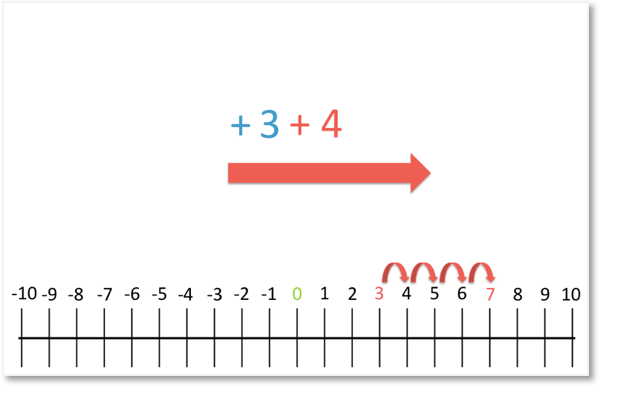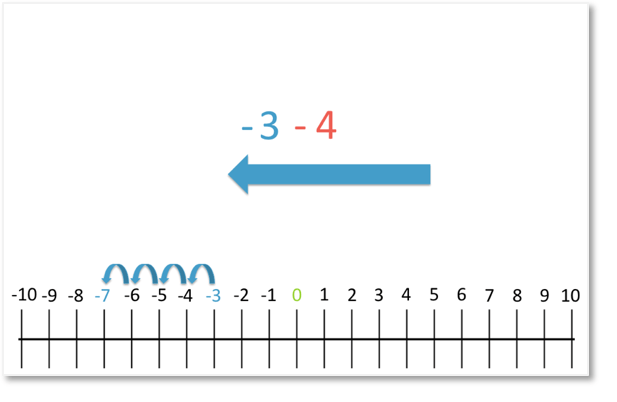# How to Subtract Positives from Negatives Using a Number Line

How to Subtract Positives from Negatives Using a Number Line

Supporting Lessons

## How to Subtract a Positive from a Negative Number using a Number Line

Below we have the calculation ‘3 + 4’.

Both 3 and 4 are positive numbers. We can show that they are both positive by writing a plus sign in front of each number.

However, plus signs are not necessary to show that a number is positive.

It is assumed that a number is positive unless a minus sign is written in front of it.The plus sign means that we move in the positive direction, which is to the right on the number line.

The ‘+3’ means that we start at 0 and move three places to the right.The ‘+4’ means that we move another four places to the right.

We have moved a total of seven places to the right on our number line.

So,

3 + 4 = 7

In the calculation below, we have ‘- 3 – 4’.The minus sign means that we move in the negative direction, which is to the left on the number line.

The ‘-3’ means that we start at 0 and move three places to the left.The ‘-4’ means that we move another four places to the left.

We have moved a total of seven places to the left.

So,

– 3 – 4 = – 7In both calculations, we moved a total of seven places.

The positive signs meant that we moved a total of seven places to the right.

The negative sign meant that we moved a total of seven places to the left.

We moved 3 left and 4 left to move 7 places left on our number line.error: Content is protected !!